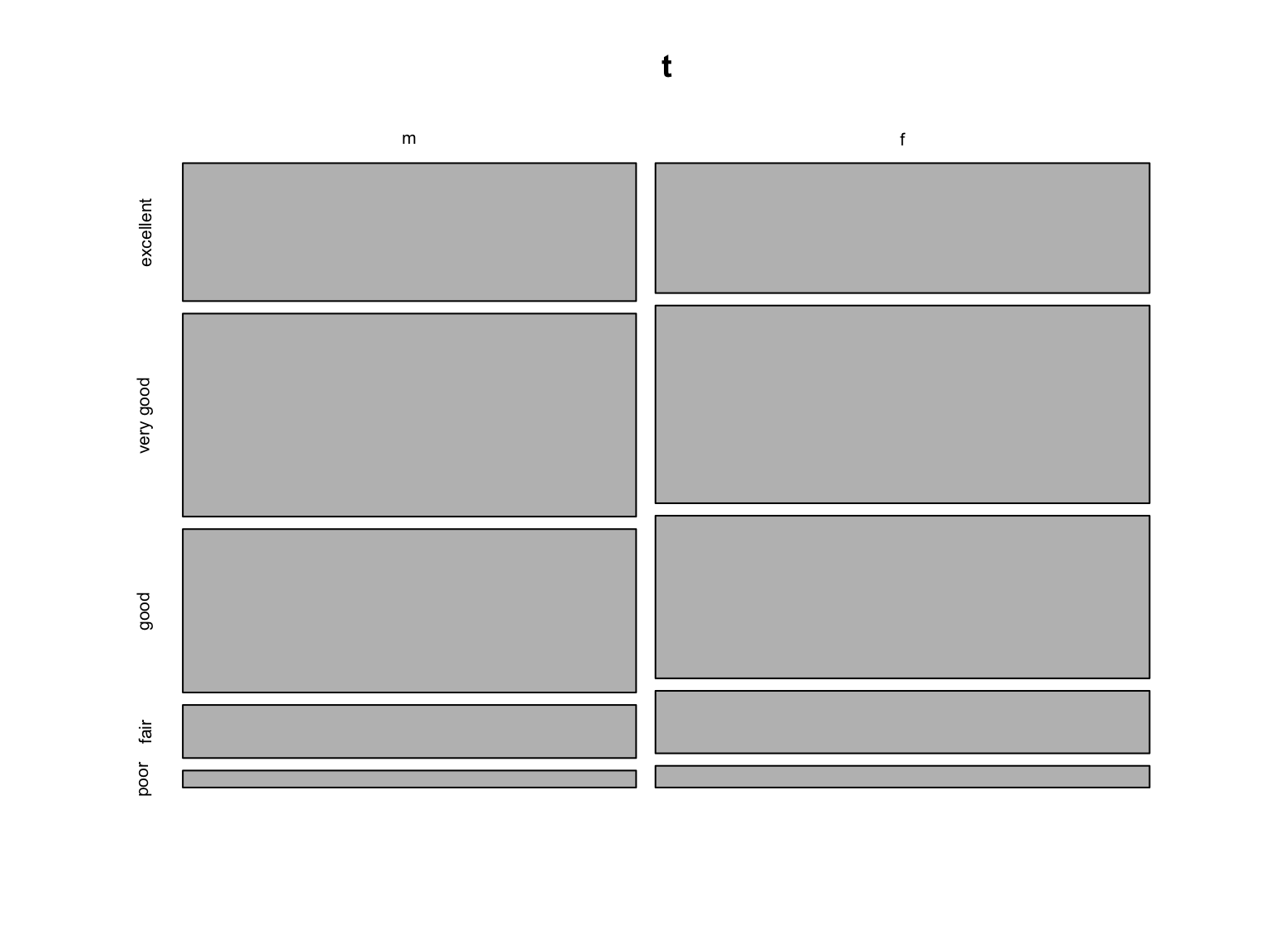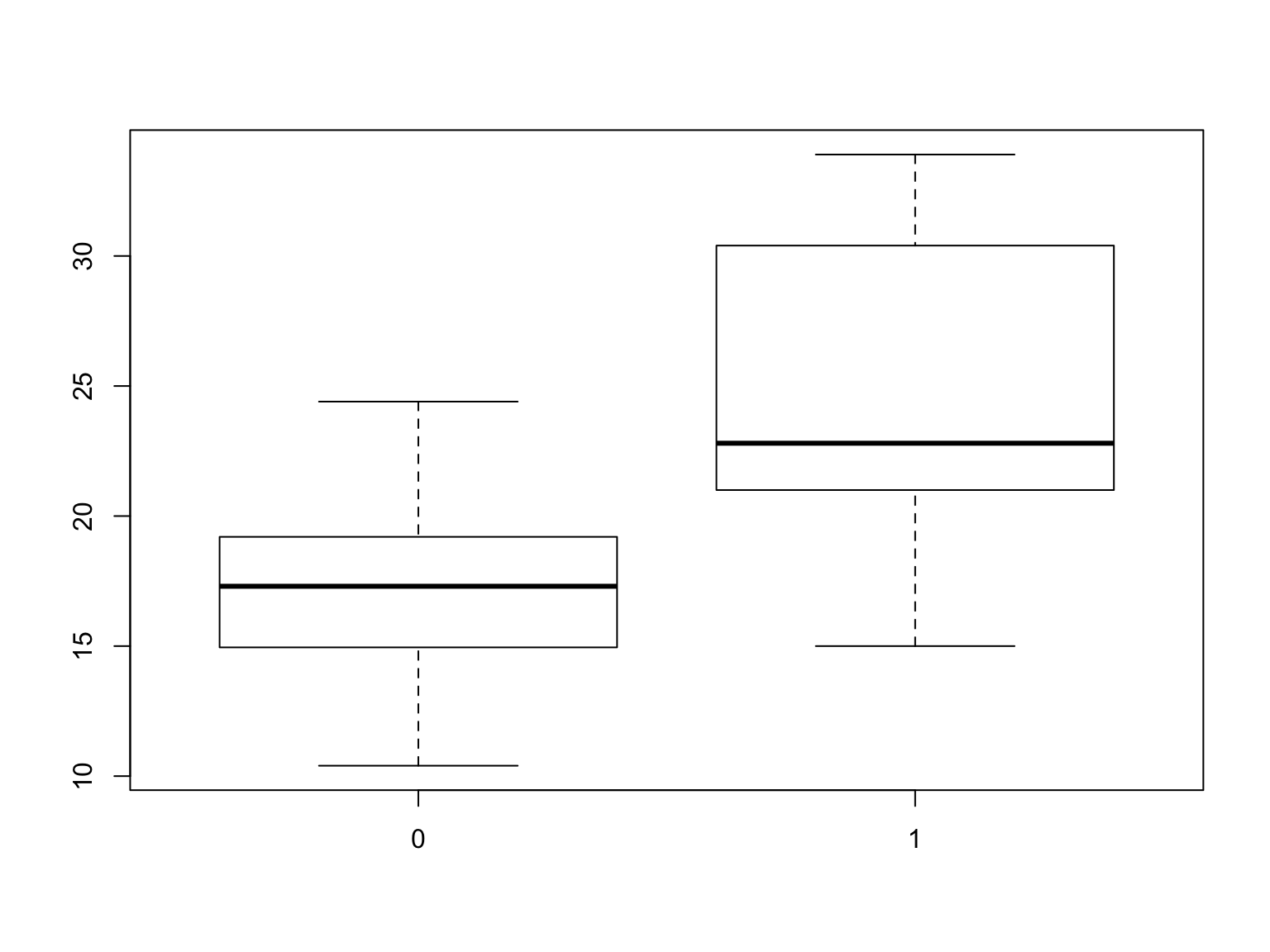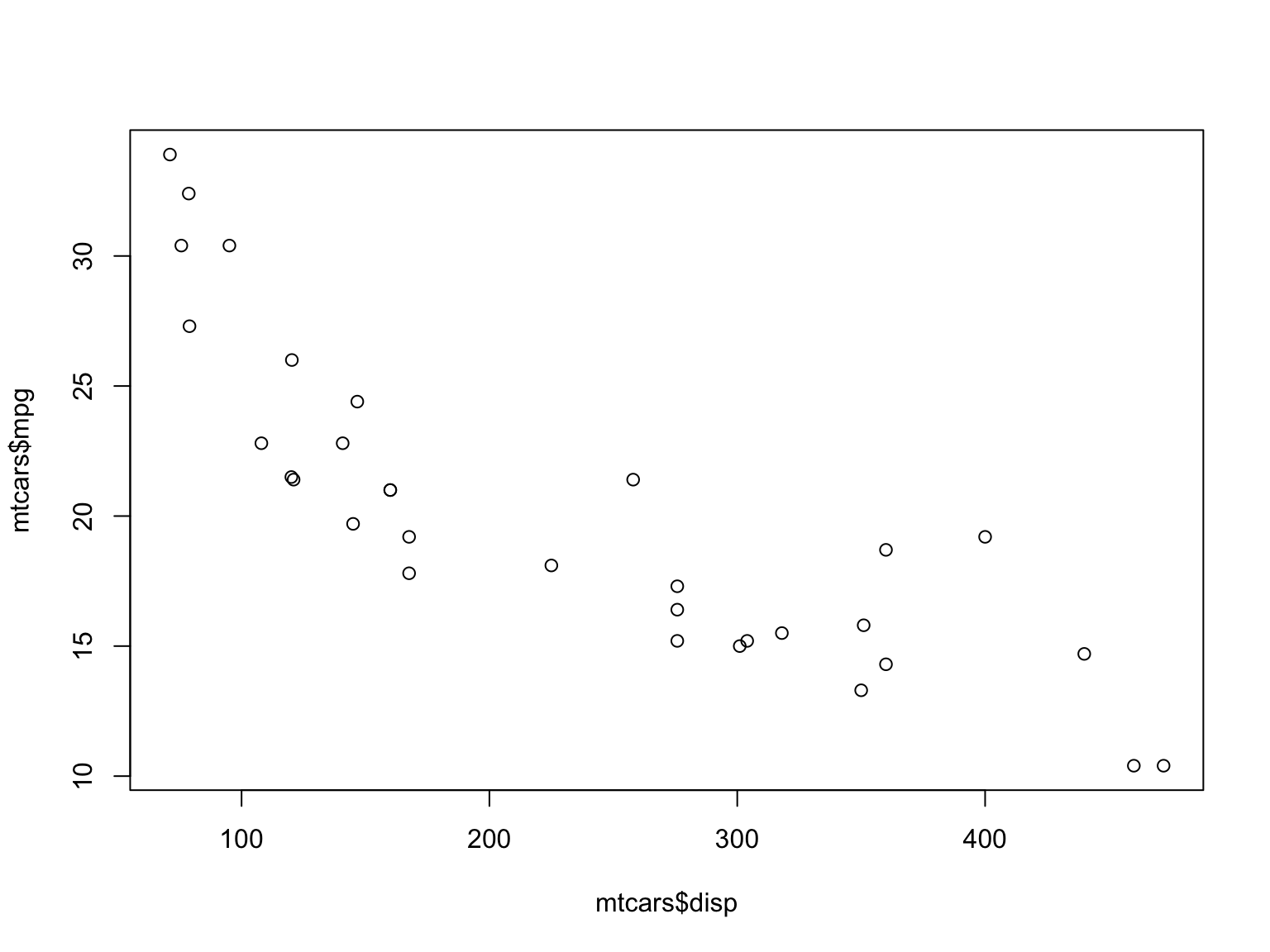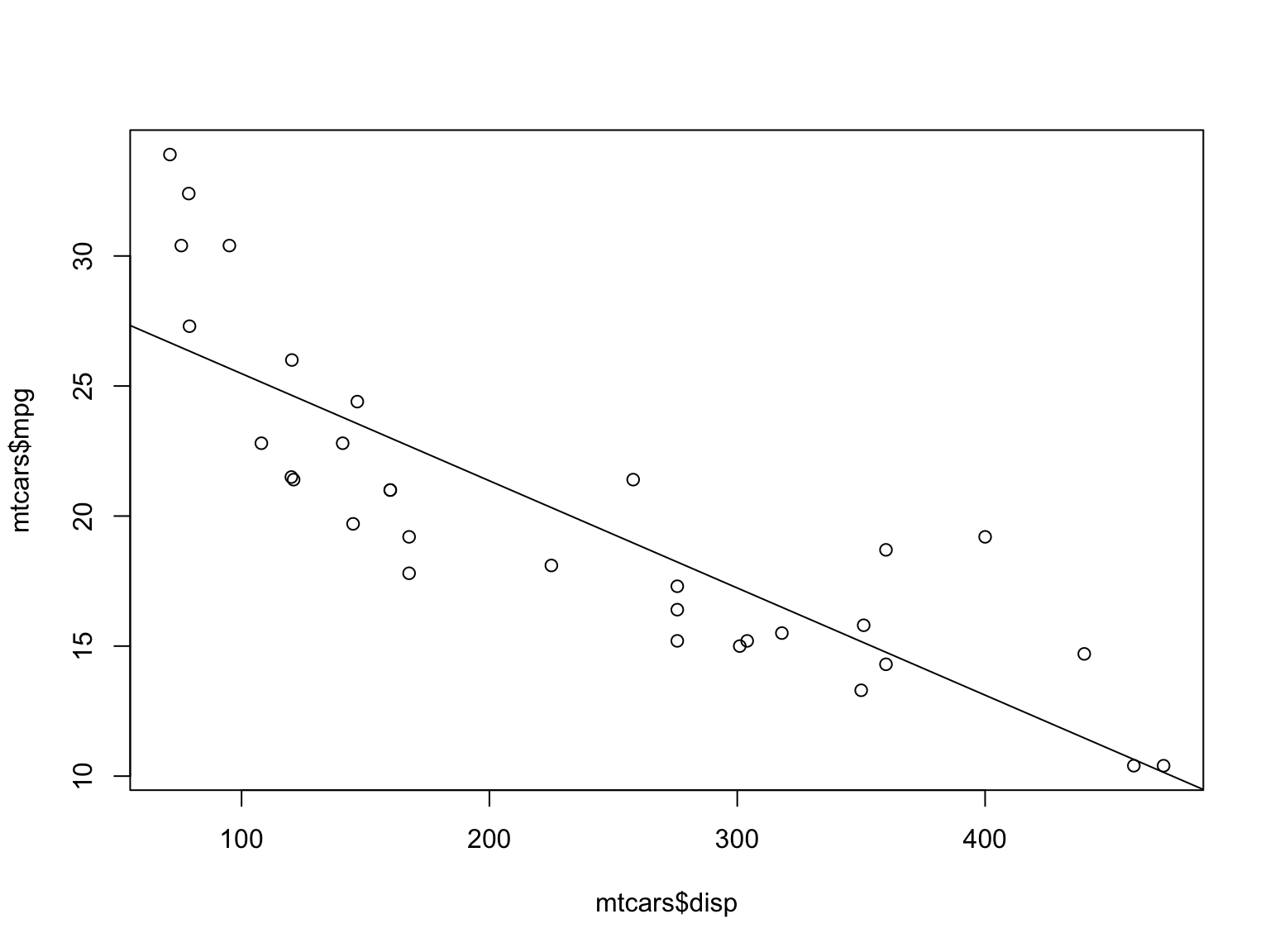10/27/2016

# Exploring Relationships Between Two Variables

### General Points

What we’re really interested in when we examine the relationship between two variables is whether or not there is a causal relationship between the two. There is usually a theory telling us that the value of one of the two variables influences the value of the other variable. The first variable is referred to as the explanatory variable and the second variable is referred to as the response. There are other pairs of terms, which may be more familiar

• Cause/Effect
• Independent/Dependent
• Input/Output
• Driver/Outcome

Whatever terms we use, there are four possible types of analysis depending on the types of the two variables.

1. Categorical Explanatory and Categorical Response
2. Categorical Explanatory and Quantitative Response
3. Quantitative Explanatory and Quantitative Response
4. Quantitaive Explanatory and Categorical Response

# Correlation and Causation

We are about to examine some graphical and numerical techniques for exploring the idea of causal relationships. We will see if there is some correlation between the variables. However we must always repeat the mantra “Correlation does not imply Causation.”

Whenever we examine data and think we should conclude that some variable A causes another variable B, we have to recoginize that there are four possibilities for what we see.

• A really does cause B.
• B really does cause A.
• C, which is unobserved, causes both A and B.
• The observed results are simply the result of random fluctuations.

# Two Categorical Variables

Let’s look at the relationship between gender and genhlth in the cdc dataset. First we need to cdc dataframe.

load("~/cdc.Rdata")

The table() command in R gives us the basic numerical results.

table(cdc$gender,cdc$genhlth)
##
##     excellent very good good fair poor
##   m      2298      3382 2722  884  283
##   f      2359      3590 2953 1135  394

# Using CrossTable

There is a much better command, CrossTable() in the gmodels package. You will need to install the gmodels package.

library(gmodels)
CrossTable(cdc$gender,cdc$genhlth)
##
##
##    Cell Contents
## |-------------------------|
## |                       N |
## | Chi-square contribution |
## |           N / Row Total |
## |           N / Col Total |
## |         N / Table Total |
## |-------------------------|
##
##
## Total Observations in Table:  20000
##
##
##              | cdc$genhlth ## cdc$gender | excellent | very good |      good |      fair |      poor | Row Total |
## -------------|-----------|-----------|-----------|-----------|-----------|-----------|
##            m |      2298 |      3382 |      2722 |       884 |       283 |      9569 |
##              |     2.190 |     0.641 |     0.017 |     6.959 |     5.167 |           |
##              |     0.240 |     0.353 |     0.284 |     0.092 |     0.030 |     0.478 |
##              |     0.493 |     0.485 |     0.480 |     0.438 |     0.418 |           |
##              |     0.115 |     0.169 |     0.136 |     0.044 |     0.014 |           |
## -------------|-----------|-----------|-----------|-----------|-----------|-----------|
##            f |      2359 |      3590 |      2953 |      1135 |       394 |     10431 |
##              |     2.009 |     0.588 |     0.016 |     6.384 |     4.740 |           |
##              |     0.226 |     0.344 |     0.283 |     0.109 |     0.038 |     0.522 |
##              |     0.507 |     0.515 |     0.520 |     0.562 |     0.582 |           |
##              |     0.118 |     0.179 |     0.148 |     0.057 |     0.020 |           |
## -------------|-----------|-----------|-----------|-----------|-----------|-----------|
## Column Total |      4657 |      6972 |      5675 |      2019 |       677 |     20000 |
##              |     0.233 |     0.349 |     0.284 |     0.101 |     0.034 |           |
## -------------|-----------|-----------|-----------|-----------|-----------|-----------|
##
## 

# The Mosaic Plot.

A mosaic plot shows the relationship between two categorical variables graphically. It is based on an existing table, and can’t be created directly from raw data.

t = table(cdc$gender,cdc$genhlth)
mosaicplot(t)# Categorical Explanatory and Quantitative Response

For this case, we’ll work with the mtcars dataframe, which is always avaialble in base R. it is included in the datasets package, where you can see some documentation.

Let’s do an str() and summary() to look at the data first.

str(mtcars)
## 'data.frame':    32 obs. of  11 variables:
##  $mpg : num 21 21 22.8 21.4 18.7 18.1 14.3 24.4 22.8 19.2 ... ##$ cyl : num  6 6 4 6 8 6 8 4 4 6 ...
##  $disp: num 160 160 108 258 360 ... ##$ hp  : num  110 110 93 110 175 105 245 62 95 123 ...
##  $drat: num 3.9 3.9 3.85 3.08 3.15 2.76 3.21 3.69 3.92 3.92 ... ##$ wt  : num  2.62 2.88 2.32 3.21 3.44 ...
##  $qsec: num 16.5 17 18.6 19.4 17 ... ##$ vs  : num  0 0 1 1 0 1 0 1 1 1 ...
##  $am : num 1 1 1 0 0 0 0 0 0 0 ... ##$ gear: num  4 4 4 3 3 3 3 4 4 4 ...
##  $carb: num 4 4 1 1 2 1 4 2 2 4 ... summary(mtcars) ## mpg cyl disp hp ## Min. :10.40 Min. :4.000 Min. : 71.1 Min. : 52.0 ## 1st Qu.:15.43 1st Qu.:4.000 1st Qu.:120.8 1st Qu.: 96.5 ## Median :19.20 Median :6.000 Median :196.3 Median :123.0 ## Mean :20.09 Mean :6.188 Mean :230.7 Mean :146.7 ## 3rd Qu.:22.80 3rd Qu.:8.000 3rd Qu.:326.0 3rd Qu.:180.0 ## Max. :33.90 Max. :8.000 Max. :472.0 Max. :335.0 ## drat wt qsec vs ## Min. :2.760 Min. :1.513 Min. :14.50 Min. :0.0000 ## 1st Qu.:3.080 1st Qu.:2.581 1st Qu.:16.89 1st Qu.:0.0000 ## Median :3.695 Median :3.325 Median :17.71 Median :0.0000 ## Mean :3.597 Mean :3.217 Mean :17.85 Mean :0.4375 ## 3rd Qu.:3.920 3rd Qu.:3.610 3rd Qu.:18.90 3rd Qu.:1.0000 ## Max. :4.930 Max. :5.424 Max. :22.90 Max. :1.0000 ## am gear carb ## Min. :0.0000 Min. :3.000 Min. :1.000 ## 1st Qu.:0.0000 1st Qu.:3.000 1st Qu.:2.000 ## Median :0.0000 Median :4.000 Median :2.000 ## Mean :0.4062 Mean :3.688 Mean :2.812 ## 3rd Qu.:1.0000 3rd Qu.:4.000 3rd Qu.:4.000 ## Max. :1.0000 Max. :5.000 Max. :8.000 We have a categorical variable (even though it’s coded numerically), am, which is 0 f the vehicle has an automatic transmission and 1 if the vehicle has a manual transmission. For a related quantitative variable we’ll use mpg. What we’re doing is asking if cars with stick shifts get better gas mileage than those with automatic transmissions. Now let’s use the tapply() and summary() functions together to get the numerical results. tapply(mtcars$mpg,mtcars$am,summary) ##$0
##    Min. 1st Qu.  Median    Mean 3rd Qu.    Max.
##   10.40   14.95   17.30   17.15   19.20   24.40
##
## $1 ## Min. 1st Qu. Median Mean 3rd Qu. Max. ## 15.00 21.00 22.80 24.39 30.40 33.90 Judging from these numerical results, it looks like the stick shift cars do get better mileage. Now let’s look at the graphical results. boxplot(mtcars$mpg~mtcars$am)The graphical results confirm what we saw with the numerical results. Now it’s your turn. Use the mtcars dataframe and see if the number of cylinders (cyl) influences mpg. What do you think? After you’re done, click here to see my analysis. # Quantitative Explanatory and Quantitative Response We’ll stick with the mtcars dataset since it has many numerical variables that might be related. I suspect that engines with larger displacemnts get poorer gas mileage. First, we’ll do a scatterplot with the base plot command. The independent variable should be entered first and will be graphed on the horizontal axis. We’ll also get the correlation coefficient. So let’s do this plot(mtcars$disp,mtcars$mpg)cor(mtcars$disp,mtcars$mpg) ##  -0.8475514 The graphical and numerical results confirm our theory. In the scatterplot, the points are lower as we move to the right. The correlation coefficient is close to 1 in absolute value and it has a negative value. So, it makes sense to construct a linear model in which displacement is the independent variable and mpg is the dependent variable. The general form of this model is given by $mpg = m*disp+b$ Note that I have dropped the prefix mtcars$, since we are not submitting the written equation to R.

This is the general form of what we want. Given values of $$m$$ and $$b$$, we would have a formula we could use to predict a car’s mpg if we knew its engine displacement. There is a procedure in R that will give us estimates of these two numbers based on the data we have. The function lm() produces linear models. We’ll create a linear model, named Sally and then we’ll use summary() to see the details of Sally. I’m using a familiar name jut to make the point that you can name an R object anything you want, as long as you stay within the rules.

Sally = lm(mtcars$mpg~mtcars$disp)
summary(Sally)
##
## Call:
## lm(formula = mtcars$mpg ~ mtcars$disp)
##
## Residuals:
##     Min      1Q  Median      3Q     Max
## -4.8922 -2.2022 -0.9631  1.6272  7.2305
##
## Coefficients:
##              Estimate Std. Error t value Pr(>|t|)
## (Intercept) 29.599855   1.229720  24.070  < 2e-16 ***
## mtcars$disp -0.041215 0.004712 -8.747 9.38e-10 *** ## --- ## Signif. codes: 0 '***' 0.001 '**' 0.01 '*' 0.05 '.' 0.1 ' ' 1 ## ## Residual standard error: 3.251 on 30 degrees of freedom ## Multiple R-squared: 0.7183, Adjusted R-squared: 0.709 ## F-statistic: 76.51 on 1 and 30 DF, p-value: 9.38e-10 The values of $$m$$ and $$b$$ are in the column labeled Estimate. $$b=29.599855$$ and $$m=-0.041215$$. Let’s store these values under these names in our R workspace for convenience. Then we can use them to calculate predict mpg values for different values of disp. let’s see what happens when we predict the value of mpg for two different displacement values, 200 and 201. The change in displacement between these two is only one cubic inch. b=29.599855 m=-0.041215 mpg200 = m * 200 + b mpg201 = m * 201 + b delta.mpg = mpg201 - mpg200 delta.mpg ##  -0.041215 There are a few points to be made based on these computations. 1. $$b$$ is the intercept, the predicted value of mpg when disp = 0. 2. The slope is the value of $$m$$. Note that when we increased engine displacement by $$1$$, from $$200$$ to $$201$$, the mpg decreased by $$m$$. This is exactly what we expected to see. We can add the actual line to the scatterplot with the abline command. Note that we need to re-run the plot command right before we run the abline command. Also note that we used a tilde, “~” rather than a comma, “,” between the two variables in the plot command. This alternative puts the explanatory and response variables in the same place in the plot command as in the lm command. plot(mtcars$mpg~mtcars\$disp)
abline(Sally)Note how the line does seem to fit the points. Another point to make is that the lower left corner in the plot display is not (0,0). It has a y-coordinate of about 10 and an x-coordinate of about 75. That explains why the plotted line does not intercept the apparent vertical axis at the value given by the intercept in the linear model output.

Now it’s your turn again. use the tools explained above to investigate the relationship between the weight of the vehicle and the gas mileage. When you’re done click here to see how I would do this.